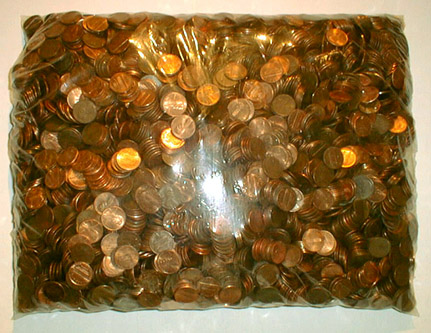to japanese | index | next

US\$63.68 Which Consists of 6232 Coins (Money Amount No. 30)

The U. S. coins, a plastic bag
2002

cf. 6232 and 6368 are the fourth smallest amicable pair which Euler found in 1750.
(Two numbers are called amicable if each equals to the sum of the divisors of the other.)
Divisors of 6232: 1 + 2 + 4 + 8 + 19 + 38 + 41 + 76 + 82 + 152 + 164 + 328 + 779 + 1558 + 3116 = 6368
Divisors of 6368: 1 + 2 + 4 + 8 + 16 + 32 + 199 + 398 + 796 + 1592 + 3184 = 6232